# Test: Area Of Parallelograms

## 10 Questions MCQ Test Mathematics (Maths) Class 9 | Test: Area Of Parallelograms

Description
This mock test of Test: Area Of Parallelograms for Class 9 helps you for every Class 9 entrance exam. This contains 10 Multiple Choice Questions for Class 9 Test: Area Of Parallelograms (mcq) to study with solutions a complete question bank. The solved questions answers in this Test: Area Of Parallelograms quiz give you a good mix of easy questions and tough questions. Class 9 students definitely take this Test: Area Of Parallelograms exercise for a better result in the exam. You can find other Test: Area Of Parallelograms extra questions, long questions & short questions for Class 9 on EduRev as well by searching above.
QUESTION: 1

### Two parallelograms are on equal bases and between the same parallels. The ratio of their areas is:

Solution:

Two parallelograms are on equal bases and between the same parallels.
i.e., their corresponding heights must be the same.
Thus, areas of both parallelograms are equal. Hence, the ratio of their areas is 1:1.

QUESTION: 2

###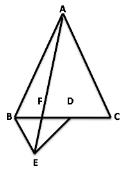In the figure, ABC and BDE are two equilateral triangles such that D is the midpoint of BC. If AE intersects BC at F, then

Solution:

Let the altitude of the triangle ABC is h

Then altitude of the triangle BED = h/2

Now, ar(Δ FED) = (1/2)*FD*(h/2) = (FD  * h)/2

and ar(Δ AFC) = (1/2)*FC*h

= (1/2)*(FD + DC)*h

= (1/2)*(FD + BD)*h

= (1/2)*(FD + BF +  FD)*h

= (1/2)*(2FD + BF)*h

= (1/2)*(2FD + 2FD)*h

= 2FD*H

⇒  ar(Δ AFC)/8 = 2FD*H/8

⇒  ar(Δ AFC)/8 = 2FD*H/4

⇒  ar(Δ AFC)/8 = ar(Δ FED)

QUESTION: 3

### In parallelogram ABCD, AL ⊥ CD and CM ⊥ AD. If AL=20cm, CD = 12 cm, CM=8cm, then perimeter of the parallelogram is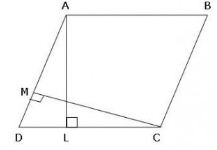Solution:

Given that ABCD is a IIgm

AL = 20 cm

CD = 12 cm

CM = 8 cm

Area of IIgm ABCD will be = BASE * ALTITUDE

= AL * CD

= 20 * 12= 240 cm2

Again taking base AD and Altitude MC

than Ar IIgm ABCD = B * h

or  AD = 240/8 = 30 cm

now ,

CD = AB = 12 cm (opp. sides of a IIgm)

AD = BC = 30 cm(opp. sides of a IIgm)

now ,

Perimeter of IIgm ABCD =

2a + 2b

or 2(a+b)

2 (30+12)

2(42)

or, 42*2 = 84 cm

QUESTION: 4

PQRS is a trapezium in which PQ = 7 cm, PS = QR = 5 cm, RS = x cm and distance between PQ and RS is 4 cm. The area of trapezium is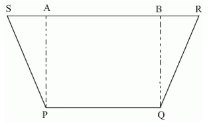Solution:
QUESTION: 5

A triangle and a rhombus are on the same base and between the same parallels. Then the ratio of area of triangle to that rhombus is:

Solution:

If a triangle and a rhombus are on the same base and between the same parallels then the ratio of the areas of the triangle and rhombus is 1 : 2

The base of triangle and rhombus are equal. The rhombus have equal sides, and  one side is the base of triangle.

The are of triangle must be half of the are of rhombus.

QUESTION: 6

ABCD is a parallelogram of area 100 cm2. AE is drawn perpendicular to BC and AF is drawn perpendicular to DC. If AE = 8 cm and AF is equal to 6.25 cm, then AB is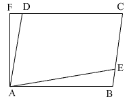Solution:

Area = B x H
100 = DC x AF
100 = DC x 6.25
DC = 100/6.25
DC = 16cm
AB = DC( in a parallelogram opposite sides are equal )

So AB = 16cm

QUESTION: 7

Parallelogram ABCD and rectangle ABEF are on the same base AB. If AB = 14 cm, BC = 12 cm, then the possible value for the perimeter of ABEF is

Solution:

IIgm ABCD and rectangle ABEF are between the same parallels AB and CF.
AB = EF (For rectangle) and AB = CD (For parallelogram)
? CD = EF ? AB + CD = AB + EF ... (1)
Of all the line segments that can be drawn to a given line from a point not lying on it, the perpendicular line segment is the shortest.
similarly we write , BE < BC ? AF + BE < AD + BC ... (2)
From equations (1) and (2), we get
AB + EF + AF + BE < AD + BC + AB + CD
Perimeter of rectangle ABEF < Perimeter of parallelogram ABCD

Now,  Perimeter of parallelogram ABCD = AB + BC + CD + DA = 14 + 12 + 14 + 12 = 52

Thus, Perimeter of rectangle ABEF < 52 cm

QUESTION: 8

ABCD is a parallelogram, Area(DAB) =

Solution:

ABCD is a parallelogram
In a Triangle ABC and ADC
AB=CD(opposite lines of a parallelogram)
AC=AC(common)
Triangle ABC and ADC ( SSS Cong. rule)
Hence proved.

QUESTION: 9

In the following figure, if ED = 10 cm, BG = 8 cm and BE = CD = 9 cm, then find the area of triangle CDF.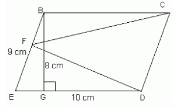Solution:

If a triangle and a parallelogram are on the same base and between the same parallels, then the area of the triangle is equal to half the area of the parallelogram.

Here, the area of the parallelogram = base x height = 10 x 8 = 80 cm2

Therefore, the area of the triangle = 1/2 x area of parallelogram

= 1/2 x 80 = 40 cm2

QUESTION: 10

Find the area of quadrilateral ABCD, if AB || CD and AB = CD is as shown in figure :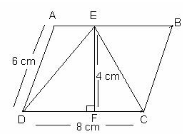Solution:

This is a condition of parallelogram

Area = EF×DC =4  cm × 8 cm = 32 sq. cm